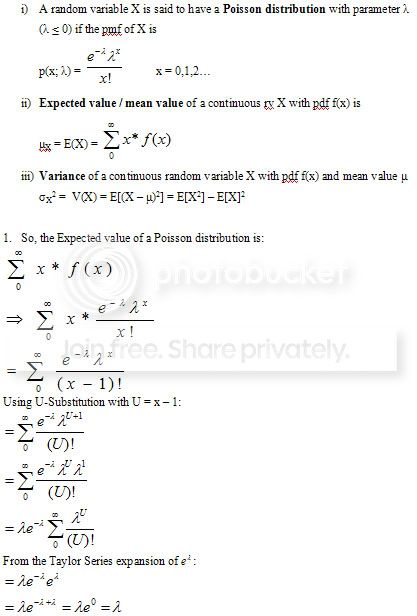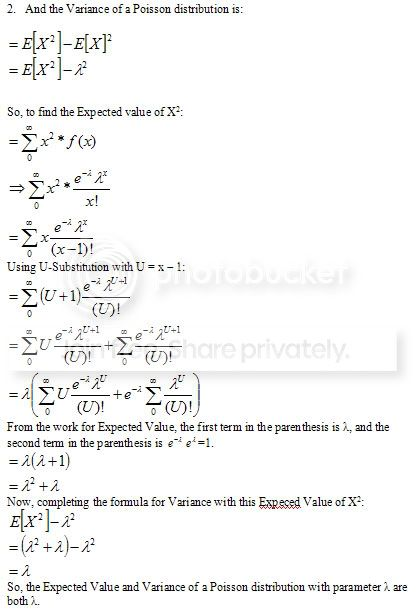# Math geeks: Probability question

#### alan

##### Distinguished Member
What is the proof that the Expected value and Variance of a Poisson variable with parameter &quot;m&quot; are &quot;m&quot; itself?

#### rdawson808

##### Distinguished Member
Serious? No clue. But I do know that my Mathematician and Statistician friends would be mad that you're confusing the two. Mods, change the title to "Stats geeks:..."

Have you checked a stats book? An advanced Prob. and Dist. Theory one?

Actually, it shouldn't be that hard, should it?

You know the formula for expected value of a random variable

E[m] = integral of mf(m) wrt m

And you know the formula for variance of a random variable

Var(m) = integral of (m-mu)^2f(m)dm or you can use Var(m) = E[m^2] - mu^2.

so if your contention is that both are equal to m, then set them equal and solve them. If they don't come out equal, haven't you proven it? I guess that'd only prove that they are equal to one another, not that they're both equal to m. but at least if you show they are equal then you only have to prove that one of them equals m, which could make life easier. Hmmm. I never was good at stats.

b

#### jgold47

##### Distinguished Member
Serious? No clue. But I do know that my Mathematician and Statistician friends would be mad that you're confusing the two. Mods, change the title to "Stats geeks:..."

Have you checked a stats book? An advanced Prob. and Dist. Theory one?

Actually, it shouldn't be that hard, should it?

You know the formula for expected value of a random variable

E[m] = integral of mf(m) wrt m

And you know the formula for variance of a random variable

Var(m) = integral of (m-mu)^2f(m)dm or you can use Var(m) = E[m^2] - mu^2.

so if your contention is that both are equal to m, then set them equal and solve them. If they don't come out equal, haven't you proven it? I guess that'd only prove that they are equal to one another, not that they're both equal to m. but at least if you show they are equal then you only have to prove that one of them equals m, which could make life easier. Hmmm. I never was good at stats.

b

F you for knowing that. Now go slam yourself into a locker and give me your lunch money.

#### feynmix

##### Distinguished Member
Since the poisson distribution is discrete, the proof isn't trivial. You just can't do the integral. You are gonna have to do the summations, and there is probably a summation trick or two in there to get it all looking nice and elegant. Set up the definition of expected value and variance and you will see this.

Also, for a math proof like this, you can't set them equal to each other. You solve each one of them individually, and show that the result is the same.

#### rdawson808

##### Distinguished Member
Since the poisson distribution is discrete, the proof isn't trivial. You just can't do the integral. You are gonna have to do the summations, and there is probably a summation trick or two in there to get it all looking nice and elegant. Set up the definition of expected value and variance and you will see this.

Ah, I wasn't sure if it was discrete or continuous.

Also, for a math proof like this, you can't set them equal to each other. You solve each one of them individually, and show that the result is the same.

Sure, your way works, but mine might be easier--and also works. If it's easier to prove they are equal to one another, and then just find the value of one rather than prove the values of both are the same, then do the easier thing. And it looks like setting them equal allows you do eliminate a bunch of crap off the bat. It may not be easier, but it seems valid.

b

#### unjung

##### Distinguished Member^^ thank you.

#### feynmix

##### Distinguished Member

Sure, your way works, but mine might be easier--and also works. If it's easier to prove they are equal to one another, and then just find the value of one rather than prove the values of both are the same, then do the easier thing. And it looks like setting them equal allows you do eliminate a bunch of crap off the bat. It may not be easier, but it seems valid.

b

In this case, you need to find the find the mean in order to find the variance. And since the terms are sitting inside the summation, nothing will cancel.Also, your way definitely works too, its just less applicable in a lot of cases. Another way of looking at your way is that the difference between the two is zero, thats how your way is normally started.

e=mc^2

#### rdawson808

##### Distinguished Member
In this case, you need to find the find the mean in order to find the variance. And since the terms are sitting inside the summation, nothing will cancel.Also, your way definitely works too, its just less applicable in a lot of cases. Another way of looking at your way is that the difference between the two is zero, thats how your way is normally started.

Damnit! I was never good at finding those tricks to making my life easier in math and stats classes. That's so frustrating when your prof (or a classmate) says, "Oh but if you remember, you can ______________ and then that simplifies things and it only takes a minute," rather than the hour I spent on it.

b

#### alan

##### Distinguished Member
rdawson i think your way is wrong but thats because i may have screwed up the original post.

Wingedmongoose posted a page which has the solution and its right. I forgot my taylor series so wouldnt have come up with that one.

As for the mistake, when i said parameter m, it doesnt mean the discrete variable is m, its X, but has parameter m, such that f(x)= e^(-m).m^(x)/x!

#### rdawson808

##### Distinguished Member
rdawson i think your way is wrong but thats because i may have screwed up the original post.

Wingedmongoose posted a page which has the solution and its right. I forgot my taylor series so wouldnt have come up with that one.

As for the mistake, when i said parameter m, it doesnt mean the discrete variable is m, its X, but has parameter m, such that f(x)= e^(-m).m^(x)/x!

Yeah, that threw me off. And proves it's just a good thing I dind't get that job at PLU that would have included teaching intro econ stats.

b

#### DBoon

##### Senior MemberEDIT: When I defined the pdf of a Poisson distribution, it should be "lambda is greater than 0". And, apparently someone already provided a link to the solution ...well, shit I'm taking Statistics for Engineers so solving this wasn't totally useless## LARGE METAL WATCHES WITH TAILORING

• ### No watch at all.

Results are only viewable after voting.